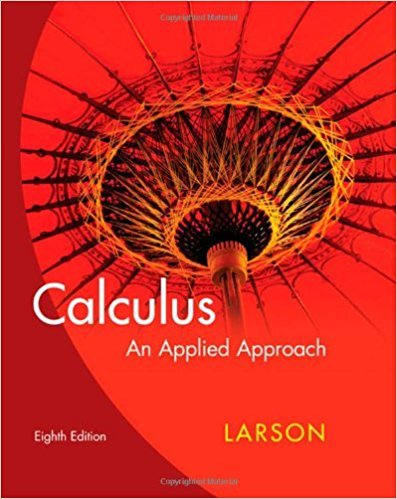×
×

# Solutions for Chapter 5.3: Exponential and Logarithmic Integrals## Full solutions for Calculus: An Applied Approach | 8th Edition

ISBN: 9780618958252Solutions for Chapter 5.3: Exponential and Logarithmic Integrals

Solutions for Chapter 5.3
4 5 0 374 Reviews
10
0
##### ISBN: 9780618958252

Since 64 problems in chapter 5.3: Exponential and Logarithmic Integrals have been answered, more than 23991 students have viewed full step-by-step solutions from this chapter. This expansive textbook survival guide covers the following chapters and their solutions. Calculus: An Applied Approach was written by and is associated to the ISBN: 9780618958252. Chapter 5.3: Exponential and Logarithmic Integrals includes 64 full step-by-step solutions. This textbook survival guide was created for the textbook: Calculus: An Applied Approach , edition: 8.

Key Calculus Terms and definitions covered in this textbook
• Additive inverse of a complex number

The opposite of a + bi, or -a - bi

• Algebraic expression

A combination of variables and constants involving addition, subtraction, multiplication, division, powers, and roots

• Annual percentage yield (APY)

The rate that would give the same return if interest were computed just once a year

• Compounded annually

See Compounded k times per year.

• Compounded continuously

Interest compounded using the formula A = Pert

• Constraints

See Linear programming problem.

• Data

Facts collected for statistical purposes (singular form is datum)

• Definite integral

The definite integral of the function ƒ over [a,b] is Lbaƒ(x) dx = limn: q ani=1 ƒ(xi) ¢x provided the limit of the Riemann sums exists

• Exponential decay function

Decay modeled by ƒ(x) = a ? bx, a > 0 with 0 < b < 1.

• Exponential function

A function of the form ƒ(x) = a ? bx,where ?0, b > 0 b ?1

• Half-angle identity

Identity involving a trigonometric function of u/2.

• Imaginary axis

See Complex plane.

• Instantaneous rate of change

See Derivative at x = a.

A measure that tells how widely distributed data are.

• Obtuse triangle

A triangle in which one angle is greater than 90°.

• Parametric equations

Equations of the form x = ƒ(t) and y = g(t) for all t in an interval I. The variable t is the parameter and I is the parameter interval.

• Reduced row echelon form

A matrix in row echelon form with every column that has a leading 1 having 0’s in all other positions.

• Row echelon form

A matrix in which rows consisting of all 0’s occur only at the bottom of the matrix, the first nonzero entry in any row with nonzero entries is 1, and the leading 1’s move to the right as we move down the rows.

• Scientific notation

A positive number written as c x 10m, where 1 ? c < 10 and m is an integer.

• Tangent

The function y = tan x

×